Courses

# Test: Metal Casting - 2

## 30 Questions MCQ Test RRB JE for Mechanical Engineering | Test: Metal Casting - 2

Description
This mock test of Test: Metal Casting - 2 for Mechanical Engineering helps you for every Mechanical Engineering entrance exam. This contains 30 Multiple Choice Questions for Mechanical Engineering Test: Metal Casting - 2 (mcq) to study with solutions a complete question bank. The solved questions answers in this Test: Metal Casting - 2 quiz give you a good mix of easy questions and tough questions. Mechanical Engineering students definitely take this Test: Metal Casting - 2 exercise for a better result in the exam. You can find other Test: Metal Casting - 2 extra questions, long questions & short questions for Mechanical Engineering on EduRev as well by searching above.
QUESTION: 1

Solution:
QUESTION: 2

Solution:
QUESTION: 3

### Which of the following materials requires the largest shrinkage allowance, while making a pattern for casting?

Solution:

Shrinkage allowance is given by,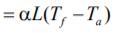Where, α = Coefficient of thermal expansion.
So, Shrinkage ∝ α
Since, brass has the highest coefficient of
thermal expansion among the given materials
so, it will require largest shrinkage allowance.
Hence, the correct option is (B).

QUESTION: 4

Which of the following pattern-materials are used in Precision Casting?

1. Plaster of Paris

2. Plastics

3. Anodized Aluminium Alloy

4. Frozen Mercury

Select the correct answer using the codes given below:

Solution:
QUESTION: 5

Gray cast iron blocks 200 x 100 x 10 mm are to be cast in sand moulds. Shrinkage allowance for pattern making is 1%. The ratio of the volume of pattern to that of the casting will be

Solution:

Given: Dimension of block = 200 x 100 x 10 mm
Shrinkage allowance, X = %
We know that, since metal shrinks on solidification and contracts further on cooling to room temperature, linear dimensions of patterns are increased in respect of those of the finished casting to be obtained.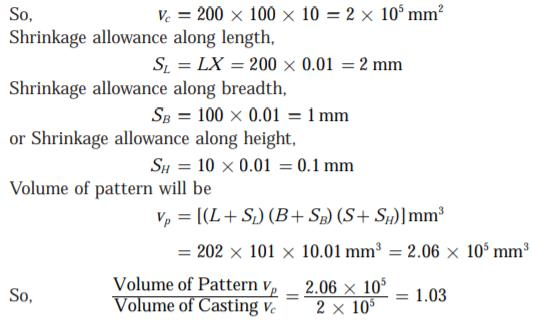QUESTION: 6

Assertion (A): In CO2 casting process, the mould or core attains maximum strength.

Reason (R): The optimum gassing time of CO2 through the mould or core forms Silica Gel which imparts sufficient strength to the mould or core.

Solution:
QUESTION: 7

Hardness of green sand mould increases with

Solution:
QUESTION: 8

In gating system design, which one of the following is the correct sequence in which choke area, pouring time, pouring basin and sprue sizes are calculated?

Solution:
QUESTION: 9

Which of the following are the requirements of an ideal gating system?

1. The molten metal should enter the mould cavity with as high a velocity as possible

2. It should facilitate complete filling of the mould cavity.

3. It should be able to prevent the absorption of air or gases from the surroundings on the molten metal while flowing through it.

Select the correct answer using the codes given below:

Solution:
QUESTION: 10

A sand casting mould assembly IS shown in the above figure. The elements marked A and B are respectively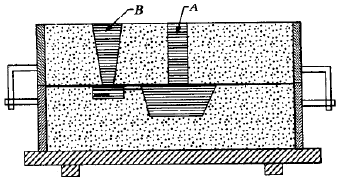Solution:
QUESTION: 11

A spherical drop of molten metal of radius 2 mm was found to solidify in 10 seconds. A similar drop of radius 4 mm would solidify in

Solution: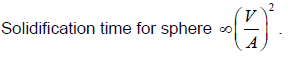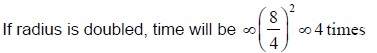QUESTION: 12

In a sand casting operation, the total liquid head is maintained constant such that it is equal to the mould height. The time taken to fill the mould with a top gate is tA. If the same mould is filled with a bottom gate, then the time taken is tB. Ignore the time required to fill the runner and frictional effects. Assume atmospheric pressure at the top molten metal surfaces. The relation between tA and tB is

Solution: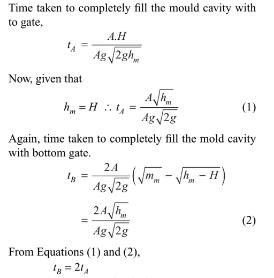QUESTION: 13

Chills are used in casting moulds to

Solution:
QUESTION: 14

Directional solidification can be achieved by providing

Solution:
QUESTION: 15

Two streams of liquid metal which are not hot enough to fuse properly result into a casting defect known as

Solution:
QUESTION: 16

Shell moulding can be used for:

Solution:
QUESTION: 17

Consider the following ingredients used in moulding:

1. Dry silica sand

2. Clay

3. Phenol formaldehyde

4. Sodium silicate

Those used for shell mould casting include

Solution:
QUESTION: 18

Consider the following statements in respect of investment castings:

1. The pattern or patterns is/are not joined to a stalk or sprue also  of wax to form a tree of patterns.

2. The prepared moulds are placed in an oven and heated gently to dry off the invest and melt out the bulk of wax.

3. The moulds are usually poured by placing the moulds in a vacuum chamber.

Which of the statements given above are correct?

Solution:

The pattern or patterns is/are joined to a stalk or sprue also of wax to form a tree of patterns.

QUESTION: 19

Which of the following pairs are correctly matched?

1. Pit moulding ... For large jobs.

2. Investment moulding ... Lost wax process.

3. Plaster moulding ... Mould prepared in gypsum.

Solution:
QUESTION: 20

The most preferred process for casting gas turbine blades is:

Solution:
QUESTION: 21

Which of the following are the most suitable materials for die casting?

Solution:
QUESTION: 22

Which of the following is not counted among the limitations of pressure die casting?

Solution:

Pressure die casting are used for mass production, the reason being, that equipments needed in pressure die casting are not available at low costs. The dies used in this process too are very expensive.

QUESTION: 23

Assertion (A): Aluminium alloys are cast in hot chamber die casting machine.

Reason (R): Aluminium alloys require high melting when compared to zinc alloys.

Solution:

The disadvantages of hot chamber die casting system is that it is limited to use with low-melting point metals and Aluminium alloys are not cast in hot chamber die casting machine because of high melting temperature.

QUESTION: 24

Consider the following statements:

1. Zinc die castings have low strength.

2. In the die casting process, very thin sections or complex shapes can be obtained easily.

Which of the statements given above is/are correct?

Solution:
QUESTION: 25

Match List-I (Name of the Process) with List-II (Advantage) and select the correct answer using the codes given below the lists: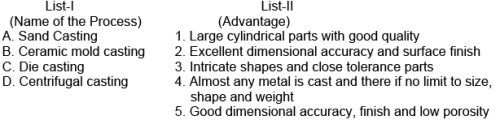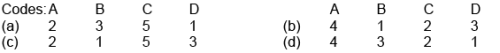Solution:

QUESTION: 26

Assertion (A): Die casting yields a product of good accuracy and finish.

Reason (R): Low melting alloys used in die casting.

Solution:
QUESTION: 27

Match the items of List I (Equipment) with the items of List II (Process) and select the correct answer using the given codes.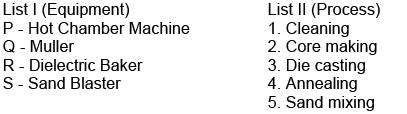Solution:
QUESTION: 28

Which one of the following is the correct statement?

In a centrifugal casting method

Solution:
QUESTION: 29

Which of the following are the most likely characteristics in centrifugal casting?

Solution: